# Tag: coalgebras

I really like Matilde Marcolli’s idea to use some of Jackson Pollock’s paintings as metaphors for noncommutative spaces. In her talk she used this painting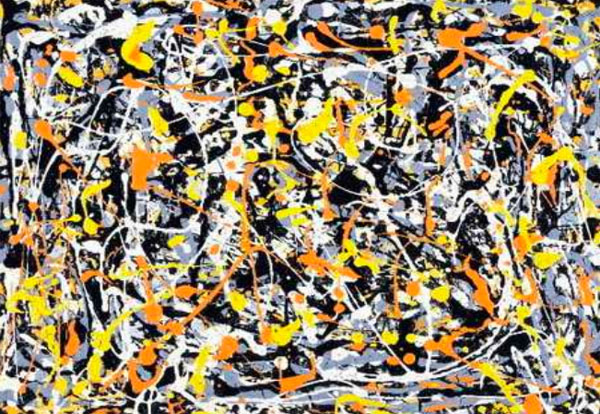and refered to it (as did I in my post) as : Jackson Pollock “Untitled N.3”. Before someone writes a post ‘The Pollock noncommutative space hoax’ (similar to my own post) let me point out that I am well aware of the controversy surrounding this painting.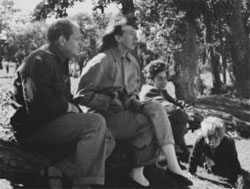This painting is among 32 works recently discovered and initially attributed to Pollock.
In fact, I’ve already told part of the story in Doodles worth millions (or not)? (thanks to PD1). The story involves the people on the right : from left to right, Jackson Pollock, his wife Lee Krasner, Mercedes Matter and her son Alex Matter.

Alex Matter, whose father, Herbert, and mother, Mercedes, were artists and friends of Jackson Pollock, discovered after his mother died a group of small drip paintings in a storage locker in Wainscott, N.Y. which he believed to be authentic Pollocks.

Read the post mentioned above if you want to know how mathematics screwed up his plan, or much better, reed the article Anatomy of the Jackson Pollock controversy by Stephen Litt.

So, perhaps the painting above was not the smartest choice, but we could take any other genuine Pollock ‘drip-painting’, a technique he taught himself towards the end of 1946 to make an image by splashing, pouring, sloshing colors onto the canvas. Typically, such a painting consists of blops of paint, connected via thin drip-lines.

What does this have to do with noncommutative geometry? Well, consider the blops as ‘points’. In commutative geometry, distinct points cannot share tangent information ((technically : a commutative semi-local ring splits as the direct sum of local rings and this does no longer hold for a noncommutative semi-local ring)). In the noncommutative world though, they can!, or if you want to phrase it like this, noncommutative points ‘can talk to each other’. And, that’s what we cherish in those drip-lines.

But then, if two points share common tangent informations, they must be awfully close to each other… so one might imagine these Pollock-lines to be strings holding these points together. Hence, it would make more sense to consider the ‘Pollock-quotient-painting’, that is, the space one gets after dividing out the relation ‘connected by drip-lines’ ((my guess is that Matilde thinks of the lines as the action of a group on the points giving a topological horrible quotient space, and thats precisely where noncommutative geometry shines)).

For this reason, my own mental picture of a genuinely noncommutative space ((that is, the variety corresponding to a huge noncommutative algebra such as free algebras, group algebras of arithmetic groups or fundamental groups)) looks more like the picture below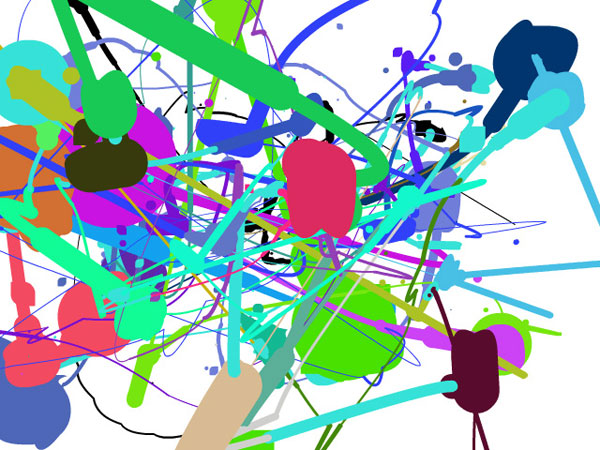The colored blops you see are really sets of points which you might view as, say, a FacebookGroup ((technically, think of them as the connected components of isomorphism classes of finite dimensional simple representations of your favorite noncommutative algebra)). Some chatter may occur between two distinct FacebookGroups, the more chatter the thicker the connection depicted ((technically, the size of the connection is the dimension of the ext-group between generic simples in the components)). Now, there are some tiny isolated spots (say blue ones in the upper right-hand quadrant). These should really be looked at as remote clusters of noncommutative points (sharing no (tangent) information whatsoever with the blops in the foregound). If we would zoom into them beyond the Planck scale (if I’m allowed to say a bollock-word in a Pollock-post) they might reveal again a whole universe similar to the interconnected blops upfront.

The picture was produced using the fabulous Pollock engine. Just use your mouse to draw and click to change colors in order to produce your very own noncommutative space!

For the mathematicians still around, this may sound like a lot of Pollock-bollocks but can be made precise. See my note Noncommutative geometry and dual coalgebras for a very terse reading. Now that coalgebras are gaining popularity, I really should write a more readable account of it, including some fanshi-wanshi examples…

Last time we tried to generalize the Connes-Consani approach to commutative algebraic geometry over the field with one element $\mathbb{F}_1$ to the noncommutative world by considering covariant functors

$N~:~\mathbf{groups} \rightarrow \mathbf{sets}$

which over $\mathbb{C}$ resp. $\mathbb{Z}$ become visible by a complex (resp. integral) algebra having suitable universal properties.

However, we didn’t specify what we meant by a complex noncommutative variety (resp. an integral noncommutative scheme). In particular, we claimed that the $\mathbb{F}_1$-‘points’ associated to the functor

$D~:~\mathbf{groups} \rightarrow \mathbf{sets} \qquad G \mapsto G_2 \times G_3$ (here $G_n$ denotes all elements of order $n$ of $G$)

were precisely the modular dessins d’enfants of Grothendieck, but didn’t give details. We’ll try to do this now.

For algebras over a field we follow the definition, due to Kontsevich and Soibelman, of so called “noncommutative thin schemes”. Actually, the thinness-condition is implicit in both Soule’s-approach as that of Connes and Consani : we do not consider R-points in general, but only those of rings R which are finite and flat over our basering (or field).

So, what is a noncommutative thin scheme anyway? Well, its a covariant functor (commuting with finite projective limits)

$\mathbb{X}~:~\mathbf{Alg}^{fd}_k \rightarrow \mathbf{sets}$

from finite-dimensional (possibly noncommutative) $k$-algebras to sets. Now, the usual dual-space operator gives an anti-equivalence of categories

$\mathbf{Alg}^{fd}_k \leftrightarrow \mathbf{Coalg}^{fd}_k \qquad A=C^* \leftrightarrow C=A^*$

so a thin scheme can also be viewed as a contra-variant functor (commuting with finite direct limits)

$\mathbb{X}~:~\mathbf{Coalg}^{fd}_k \rightarrow \mathbf{Sets}$

In particular, we are interested to associated to any {tex]k $-algebra$A $its representation functor :$\mathbf{rep}(A)~:~\mathbf{Coalg}^{fd}_k \rightarrow \mathbf{Sets} \qquad C \mapsto Alg_k(A,C^*) $This may look strange at first sight, but$C^* $is a finite dimensional algebra and any$n $-dimensional representation of$A $is an algebra map$A \rightarrow M_n(k) $and we take$C $to be the dual coalgebra of this image. Kontsevich and Soibelman proved that every noncommutative thin scheme$\mathbb{X} $is representable by a$k $-coalgebra. That is, there exists a unique coalgebra$C_{\mathbb{X}} $(which they call the coalgebra of ‘distributions’ of$\mathbb{X} $) such that for every finite dimensional$k $-algebra$B $we have$\mathbb{X}(B) = Coalg_k(B^*,C_{\mathbb{X}}) $In the case of interest to us, that is for the functor$\mathbf{rep}(A) $the coalgebra of distributions is Kostant’s dual coalgebra$A^o $. This is the not the full linear dual of$A $but contains only those linear functionals on$A $which factor through a finite dimensional quotient. So? You’ve exchanged an algebra$A $for some coalgebra$A^o $, but where’s the geometry in all this? Well, let’s look at the commutative case. Suppose$A= \mathbb{C}[X] $is the coordinate ring of a smooth affine variety$X $, then its dual coalgebra looks like$\mathbb{C}[X]^o = \oplus_{x \in X} U(T_x(X)) $the direct sum of all universal (co)algebras of tangent spaces at points$x \in X $. But how do we get the variety out of this? Well, any coalgebra has a coradical (being the sun of all simple subcoalgebras) and in the case just mentioned we have$corad(\mathbb{C}[X]^o) = \oplus_{x \in X} \mathbb{C} e_x $so every point corresponds to a unique simple component of the coradical. In the general case, the coradical of the dual coalgebra$A^o $is the direct sum of all simple finite dimensional representations of$A $. That is, the direct summands of the coalgebra give us a noncommutative variety whose points are the simple representations, and the remainder of the coalgebra of distributions accounts for infinitesimal information on these points (as do the tangent spaces in the commutative case). In fact, it was a surprise to me that one can describe the dual coalgebra quite explicitly, and that$A_{\infty} $-structures make their appearance quite naturally. See this paper if you’re in for the details on this. That settles the problem of what we mean by the noncommutative variety associated to a complex algebra. But what about the integral case? In the above, we used extensively the theory of Kostant-duality which works only for algebras over fields… Well, not quite. In the case of$\mathbb{Z} $(or more general, of Dedekind domains) one can repeat Kostant’s proof word for word provided one takes as the definition of the dual$\mathbb{Z} $-coalgebra of an algebra (which is$\mathbb{Z} $-torsion free)$A^o = { f~:~A \rightarrow \mathbb{Z}~:~A/Ker(f)~\text{is finitely generated and torsion free}~} $(over general rings there may be also variants of this duality, as in Street’s book an Quantum groups). Probably lots of people have come up with this, but the only explicit reference I have is to the first paper I’ve ever written. So, also for algebras over$\mathbb{Z} $we can define a suitable noncommutative integral scheme (the coradical approach accounts only for the maximal ideals rather than all primes, but somehow this is implicit in all approaches as we consider only thin schemes). Fine! So, we can make sense of the noncommutative geometrical objects corresponding to the group-algebras$\mathbb{C} \Gamma $and$\mathbb{Z} \Gamma $where$\Gamma = PSL_2(\mathbb{Z}) $is the modular group (the algebras corresponding to the$G \mapsto G_2 \times G_3 $-functor). But, what might be the points of the noncommutative scheme corresponding to$\mathbb{F}_1 \Gamma $??? Well, let’s continue the path cut out before. “Points” should correspond to finite dimensional “simple representations”. Hence, what are the finite dimensional simple$\mathbb{F}_1 $-representations of$\Gamma $? (Or, for that matter, of any group$G $) Here we come back to Javier’s post on this : a finite dimensional$\mathbb{F}_1 $-vectorspace is a finite set. A$\Gamma $-representation on this set (of n-elements) is a group-morphism$\Gamma \rightarrow GL_n(\mathbb{F}_1) = S_n $hence it gives a permutation representation of$\Gamma $on this set. But then, if finite dimensional$\mathbb{F}_1 $-representations of$\Gamma $are the finite permutation representations, then the simple ones are the transitive permutation representations. That is, the points of the noncommutative scheme corresponding to$\mathbb{F}_1 \Gamma $are the conjugacy classes of subgroups$H \subset \Gamma $such that$\Gamma/H $is finite. But these are exactly the modular dessins d’enfants introduced by Grothendieck as I explained a while back elsewhere (see for example this post and others in the same series).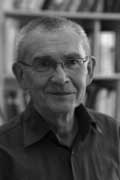Amidst all LHC-noise, Yuri I. Manin arXived today an interesting paper Cyclotomy and analytic geometry over$\mathbb{F}_1 $. The paper gives a nice survey of the existent literature and focusses on the crucial role of roots of unity in the algebraic geometry over the non-existent field with one element$\mathbb{F}_1 $(in French called ‘F-un’). I have tried to do a couple of posts on F-un some time ago but now realize, reading Manin’s paper, I may have given up way too soon… At several places in the paper, Manin hints at a possible noncommutative geometry over$\mathbb{F}_1 $: This is the appropriate place to stress that in a wider context of Toen-Vaqui ‘Au-dessous de Spec Z’, or eventually in noncommutative$\mathbb{F}_1 $-geometry, teh spectrum of$\mathbb{F}_1 $loses its privileged position as a final object of a geometric category. For example, in noncommutative geometry, or in an appropriate category of stacks, the quotient of this spectrum modulo the trivial action of a group must lie below this spectrum. Soule’s algebras$\mathcal{A}_X $are a very important element of the structure, in particular, because they form a bridge to Arakelov geometry. Soule uses concrete choices of them in order to produce ‘just right’ supply of morphisms, without a priori constraining these choices formally. In this work, we use these algebras and their version also to pave a way to the analytic (and possibly non-commutative) geometry over$\mathbb{F}_1 $. Back when I was writing the first batch of F-un posts, I briefly contemplated the possibility of a noncommutative geometry over$\mathbb{F}_1 $, but quickly forgot about it because I thought it would be forced to reduce to commutative geometry. Here is the quick argument : noncommutative geometry is really the study of coalgebras (see for example my paper or if you prefer more trustworthy sources the Kontsevich-Soibelman paper). Now, unless I made a mistake, I think all coalgebras over$\mathbb{F}_1 $must be co-commutative (even group-like), so reducing to commutative geometry. Surely, I’m missing something… Here a list of saved pdf-files of previous NeverEndingBooks-posts on geometry in reverse chronological order. Last time we saw that the _coalgebra of distributions_ of a noncommutative manifold can be described as a coalgebra Takeuchi-equivalent to the path coalgebra of a huge quiver. This infinite quiver has as its vertices the isomorphism classes of finite dimensional simple representations of the qurve A (the coordinate ring of the noncommutative manifold) and there are as many directed arrows between the vertices corresponding to the simples S and T as is the dimension of$Ext^1_A(S,T) $. The fact that this coalgebra of distributions is equivalent to the path coalgebra of _some_ quiver is in the Kontsevich-Soibelman paper though it would have been nice if they had given reference for this fact to the paper Wedge Products and Cotensor Coalgebras in Monoidal Categories by Ardizzoni or to previous work by P. Jara, D. Llena, L. Merino and D. Stefan, “Hereditary and formally smooth coalgebras”, Algebr. Represent. Theory 8 (2005), 363-374. In those papers it is shown that a coalgebra with coseparable coradical is hereditary if and only if it is formally smooth if and only if it is a cotensor coalgebra of some bicomodule. At first this looks just like the dual version of the classical result that a finite dimensional hereditary algebra is Morita equivalent to the path algebra of a quiver (which is indeed what the proof does) but again the condition that the coradical is coseparable does not require the coradical to be finite dimensional… In our case, the coradical is indeed coseparable being the direct sum over all matrix coalgebras corresponding to the simple representations. Hence, we can again recover the _points_ of our noncommutative manifold from the direct summands of the coradical. Fortunately, one can compute this huge coalgebra of distributions from a small quiver, the _one quiver to rule them all_, but as I’ve been babbling about all of this here [numerous times](http://www.neverendingbooks.org/?s=one+quiver) I’ll let the interested find out for themselves how you use it (a) to get at the isoclasses of all simples (hint : morally they are the smooth points of the quotient varieties of n-dimensional representations and enough tools have been developed recently to spot some fake simples, that is smooth proper semi-simple points) and (b) to compute the _ragball_, that is the huge quiver with vertex set the simples and arows as described above. Over the years I’ve calculated several one-quivers for a variety of qurves (such as amalgamated free products of finite groups and smooth curves). If you are in for a puzzle, try to determine it for the qurve$~(\mathbb{C}[x] \ast C_2) \ast_{\mathbb{C}
C_2} \mathbb{C} PSL_2(\mathbb{Z}) \ast_{\mathbb{C} C_3}
(\mathbb{C}[x] \ast C_3) $The answer is a mysterious hexagon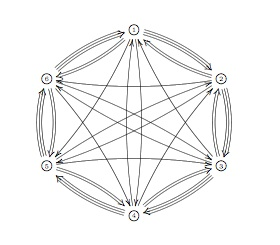Last time we have seen that the _coalgebra of distributions_ of an affine smooth variety is the direct sum (over all points) of the dual to the etale local algebras which are all of the form$\mathbb{C}[[
x_1,\ldots,x_d ]] $where$d $is the dimension of the variety. Generalizing this to _non-commutative_ manifolds, the first questions are : “What is the analogon of the power-series algebra?” and do all ‘points’ of our non-commutative manifold do have such local algebras? Surely, we no longer expect the variables to commute, so a non-commutative version of the power series algebra should be$\mathbb{C} \langle \langle x_1,\ldots,x_d \rangle \rangle $, the ring of formal power series in non-commuting variables. However, there is still another way to add non-commutativity and that is to go from an algebra to matrices over the algebra. So, in all we would expect to be our _local algebras_ at points of our non-commutative manifold to be isomorphic to$M_n(\mathbb{C} \langle \langle x_1,\ldots,x_d
\rangle \rangle) $As to the second question : _qurves_ (that is, the coordinate rings of non-commutative manifolds) do have such algebras as local rings provided we take as the ‘points’ of the non-commutative variety the set of all _simple_ finite dimensional representations of the qurve. This is a consequence of the _tubular neighborhood theorem_ due to [Cuntz](http://wwwmath.uni-muenster.de/u/cuntz/cuntz.html) and [Quillen](http://www-history.mcs.st-andrews.ac.uk/Mathematicians/Quillen.html). In more details : If A is a qurve, then a simple$n $-dimensional representation corresponds to an epimorphism$\pi~:~A \rightarrow S = M_n(\mathbb{C}) $and if we take$\mathfrak{m}=Ker(\pi) $, then$M=\mathfrak{m}/\mathfrak{m}^2 $is an$S $-bimodule and the$\mathfrak{m} $-adic completion of A is isomorphic to the completed tensor-algebra$\hat{T}_S(M) \simeq M_n(\mathbb{C}
\langle \langle x_1,\ldots,x_d \rangle \rangle) $In contrast with the commutative case however where the dimension remains constant over all points, here the numbers n and d can change from simple to simple. For n this is clear as it gives the dimension of the simple representation, but also d changes (it is the local dimension of the variety classifying simple representations of the same dimension). Here an easy example : Consider the skew group algebra$A =
\mathbb{C}[x] \star C_2 $with the action given by sending$x
\mapsto -x $. Then A is a qurve and its center is$\mathbb{C}[y] $with$y=x^2 $. Over any point$y
\not= 0 $there is a unique simple 2-dimensional representation of A giving the local algebra$M_2(\mathbb{C}[[y]]) $. If$y=0 $the situation is more complicated as the local structure of A is given by the algebra$\begin{bmatrix} \mathbb{C}[[y]] &
\mathbb{C}[[y]] \\ (y) & \mathbb{C}[[y]] \end{bmatrix} $So, over this point there are precisely 2 one-dimensional simple representations corresponding to the maximal ideals$\mathfrak{m}_1 =
\begin{bmatrix} (y) & \mathbb{C}[[y]] \\ (y) & \mathbb{C}[[y]]
\mathbb{C}[[y]] & \mathbb{C}[[y]] \\ (y) & (y) \end{bmatrix} $and both ideals are idempotent, that is$\mathfrak{m}_i^2 =
\mathfrak{m}_i $whence the corresponding bimodule$M_i =
0 $so the local algebra in either of these two points is just$\mathbb{C} $. Ok, so the comleted local algebra at each point is of the form$M_n(\mathbb{C}\langle \langle x_1,\ldots,x_d \rangle
\rangle) $, but what is the corresponding dual coalgebra. Well,$\mathbb{C} \langle \langle x_1,\ldots,x_d \rangle \rangle $is the algebra dual to the _cofree coalgebra_ on$V = \mathbb{C} x_1 +
\ldots + \mathbb{C}x_d $. As a vectorspace this is the tensor-algebra$T(V) = \mathbb{C} \langle x_1,\ldots,x_d
\rangle $with the coalgebra structure induced by the bialgebra structure defined by taking all varaibales to be primitives, that is$\Delta(x_i) = x_i \otimes 1 + 1 \otimes x_i $. That is, the coproduct on a monomial gives all different expressions$m_1 \otimes
m_2 $such that$m_1m_2 = m $. For example,$\Delta(x_1x_2) = x_1x_2 \otimes 1 + x_1 \otimes x_2 + 1 \otimes
x_1x_2 $. On the other hand, the dual coalgebra of$M_n(\mathbb{C}) $is the _matrix coalgebra_ which is the$n^2 $-dimensional vectorspace$\mathbb{C}e_{11} + \ldots +
\mathbb{C}e_{nn} $with comultiplication$\Delta(e_{ij}) =
\sum_k e_{ik} \otimes e_{kj} $The coalgebra corresponding to the local algebra$M_n(\mathbb{C}\langle \langle x_1,\ldots,x_d \rangle
\rangle) $is then the tensor-coalgebra of the matrix coalgebra and the cofree coalgebra. Having obtained the coalgebra at each point (=simple representation) of our noncommutative manifold one might think that the _coalgebra of non-commutative distributions_ should be the direct sum of all this coalgebras, summed over all points, as in the commutative case. But then we would forget about a major difference between the commutative and the non-commutative world : distinct simples can have non-trivial extensions! The mental picture one might have about simples having non-trivial extensions is that these points lie ‘infinitesimally close’ together. In the$\mathbb{C}[x] \star
C_2 $example above, the two one-dimensional simples have non-trivial extensions so they should be thought of as a cluster of two infinitesimally close points corresponding to the point$y=0 $(that is, this commutative points splits into two non-commutative points). Btw. this is the reason why non-commutative algebras can be used to resolve commutative singularities (excessive tangents can be split over several non-commutative points). While this is still pretty harmless when the algebra is finite over its center (as in the above example where only the two one-dimensionals have extensions), the situation becomes weird over general qurves as ‘usually’ distinct simples have non-trivial extensions. For example, for the free algebra$\mathbb{C}\langle x,y \rangle $this is true for all simples… So, if we want to continue using this image of points lying closely together this immediately means that non-commutative ‘affine’ manifolds behave like compact ones (in fact, it turns out to be pretty difficult to ‘glue’ together qurves into ‘bigger’ non-commutative manifolds, apart from the quiver examples of [this old paper](http://www.arxiv.org/abs/math.AG/9907136)). So, how to bring this new information into our coalgebra of distributions? Well, let’s repeat the previous argument not with just one point but with a set of finitely many points. Then we have a _semi-simple algebra_ quotient$\pi~:~A \rightarrow S = M_{n_1}(\mathbb{C}) \oplus \ldots \oplus
M_{n_k}(\mathb{C}) $and taking again$\mathfrak{m}=Ker(\pi) $and$M=\mathfrak{m}/\mathfrak{m}^2 $, then$M $is again an S-bimodule. Now, any S-bimodule can be encoded into a _quiver_ Q on k points, the number of arrows from vertex i to vertex j being the number of components in M of the form$M_{n_i \times
n_j}(\mathbb{C}) $. Again, it follows from the tubular neighborhood theorem that the$\mathfrak{m} $-adic completion of A is isomorphic to the completion of an algebra Morita equivalent to the _path algebra_$\mathbb{C} Q $(being the tensor algebra$T_S(M) $). As all the local algebras of the points are quotients of this quiver-like completion, on the coalgebra level our local coalgebras will be sub coalgebras of the coalgebra which is co-Morita equivalent (and believe it or not but coalgebraists have a name for this : _Takeuchi equivalence_) to the _quiver coalgebra_ which is the vectorspace of the path algebra$\mathbb{C} Q $with multiplication induced by making all arrows from i to j skew-primitives, that is,$\Delta(a) = e_i \otimes a + a \otimes e_j $where the$e_i $are group-likes corresponding to the vertices. If all of ths is a bit too much co to take in at once, I suggest the paper by Bill Chin [A brief introduction to coalgebra representation theory](http://condor.depaul.edu/~wchin/crt.pdf#search=%22%22A%20brief%20introduction%20to%20coalgebra%20representation%20theory%22%22). The _coalgebra of noncommutative distributions_ we are after at is now the union of all these Takeuchi-equivalent quiver coalgebras. In easy examples such as the$\mathbb{C}[x] \star C_2 $-example this coalgebra is still pretty small (the sum of the local coalgebras corresponding to the local algebras$M_2(\mathbb{C}[[x]]) $summed over all points$y \not= 0 $summed with the quiver coalgebra of the quiver$\xymatrix{\vtx{} \ar@/^/[rr] & & \vtx{}
\ar@/^/[ll]} $In general though this is a huge object and we would like to have a recipe to construct it from a manageable _blue-print_ and that is what we will do next time. In this series of posts I’ll try to make at least part of the recent [Kontsevich-Soibelman paper](http://www.arxiv.org/abs/math.RA/0606241) a bit more accessible to algebraists. In non-geometry, the algebras corresponding to *smooth affine varieties* I’ll call **qurves** (note that they are called **quasi-free algebras** by Cuntz & Quillen and **formally smooth** by Kontsevich). By definition, a qurve in an affine$\mathbb{C} $-algebra A having the lifting property for algebra maps through nilpotent ideals (extending Grothendieck’s characterization of smooth affine algebras in the commutative case). Examples of qurves are : finite dimensional semi-simple algebras (for example, group algebras$\mathbb{C} G $of finite groups), coordinate rings of smooth affine curves or a noncommutative mixture of both, skew-group algebras$\mathbb{C}[X] \ast G $whenever G is a finite group of automorphisms of the affine curve X. These are Noetherian examples but in general a qurve is quite far from being Noetherian. More typical examples of qurves are : free algebras$\mathbb{C} \langle
x_1,\ldots,x_k \rangle $and path algebras of finite quivers$~\mathbb{C} Q $. Recall that a finite quiver Q s just a directed graph and its path algebra is the vectorspace spanned by all directed paths in Q with multiplication induced by concatenation of paths. Out of these building blocks one readily constructs more involved qurves via universal algebra operations such as (amalgamated) free products, universal localizations etc. In this way, the groupalgebra of the modular group$SL_2(\mathbb{Z}) $(as well as that of a congruence subgroup) is a qurve and one can mix groups with finite groupactions on curves to get qurves like$ (\mathbb{C}[X]
\ast G) \ast_{\mathbb{C} H} \mathbb{C} M $whenever H is a common subgroup of the finite groups G and M. So we have a huge class of qurve-examples obtained from mixing finite and arithmetic groups with curves and quivers. Qurves can we used as *machines* generating interesting$A_{\infty} $-categories. Let us start by recalling some facts about finite closed subschemes of an affine smooth variety Y in the commutative case. Let **fdcom** be the category of all finite dimensional commutative$\mathbb{C} $-algebras with morphisms being onto algebra morphisms, then the study of finite closed subschemes of Y is essentially the study of the covariant functor **fdcom** –> **sets** assigning to a f.d. commutative algebra S the set of all onto algebra maps from$\mathbb{C}[Y] $to S. S being a f.d. commutative semilocal algebra is the direct sum of local factors$S
\simeq S_1 \oplus \ldots \oplus S_k $where each factor has a unique maximal ideal (a unique point in Y). Hence, our study reduces to f.d. commutative images with support in a fixed point p of Y. But all such quotients are also quotients of the completion of the local ring of Y at p which (because Y is a smooth variety, say of dimension n) is isomorphic to formal power series$~\mathbb{C}[[x_1,\ldots,x_n]] $. So the local question, at any point p of Y, reduces to finding all settings$\mathbb{C}[[x_1,\ldots,x_n]] \twoheadrightarrow S
\twoheadrightarrow \mathbb{C} $Now, we are going to do something strange (at least to an algebraist), we’re going to take duals and translate the above sequence into a coalgebra statement. Clearly, the dual$S^{\ast} $of any finite dimensional commutative algebra is a finite dimensional cocommutative coalgebra. In particular$\mathbb{C}^{\ast} \simeq \mathbb{C} $where the comultiplication makes 1 into a grouplike element, that is$\Delta(1) = 1 \otimes 1 $. As long as the (co)algebra is finite dimensional this duality works as expected : onto maps correspond to inclusions, an ideal corresponds to a sub-coalgebra a sub-algebra corresponds to a co-ideal, so in particular a local commutative algebra corresponds to an pointed irreducible cocommutative coalgebra (a coalgebra is said to be irreducible if any two non-zero subcoalgebras have non-zero intersection, it is called simple if it has no non-zero proper subcoalgebras and is called pointed if all its simple subcoalgebras are one-dimensional. But what about infinite dimensional algebras such as formal power series? Well, here the trick is not to take all dual functions but only those linear functions whose kernel contains a cofinite ideal (which brings us back to the good finite dimensional setting). If one takes only those good linear functionals, the ‘fancy’-dual$A^o $of an algebra A is indeed a coalgebra. On the other hand, the full-dual of a coalgebra is always an algebra. So, between commutative algebras and cocommutative coalgebras we have a duality by associating to an algebra its fancy-dual and to a coalgebra its full-dual (all this is explained in full detail in chapter VI of Moss Sweedler’s book ‘Hopf algebras’). So, we can dualize the above pair of onto maps to get coalgebra inclusions$\mathbb{C} \subset
S^{\ast} \subset U(\mathfrak{a}) $where the rightmost coalgebra is the coalgebra structure on the enveloping algebra of the Abelian Lie algebra of dimension n (in which all Lie-elements are primitive, that is$\Delta(x) = x \otimes 1 + 1 \otimes x $and indeed we have that$U(\mathfrak{a})^{\ast} \simeq \mathbb{C}[[x_1,\ldots,x_n]] $. We have translated our local problem to finding all f.d. subcoalgebras (containing the unique simple) of the enveloping algebra. But what is the point of this translation? Well, we are not interested in the local problem, but in the global problem, so we somehow have to **sum over all points**. Now, on the algebra level that is a problem because the sum of all local power series rings over all points is no longer an algebra, whereas the direct sum of all pointed irreducible coalgebras$~B_Y
= \oplus_{p \in Y} U(\mathfrak{a}_p) $is again a coalgebra! That is, we have found a huge coalgebra (which we call the coalgebra of ‘distributions’ on Y) such that for every f.d. commutative algebra S we have$Hom_{comm alg}(\mathbb{C}[Y],S) \simeq Hom_{cocomm
coalg}(S^{\ast},B_Y) $Can we get Y back from this coalgebra of districutions? Well, in a way, the points of Y correspond to the group-like elements, and if g is the group-like corresponding to a point p, we can recover the tangent-space at p back as the g-primitive elements of the coalgebra of distributions, that is the elements such that$\Delta(x) = x \otimes g + g \otimes x $. Observe that in this commutative case, there are no **skew-primitives**, that is elements such that$\Delta(x) = x \otimes g + h \otimes x $for different group-likes g and h. This is the coalgebra translation of the fact that a f.d. semilocal commutative algebra is the direct sum of local components. This is something that will definitely change if we try to extend the above to the case of qurves (to be continued). Here’s an appeal to the few people working in Cuntz-Quillen-Kontsevich-whoever noncommutative geometry (the one where smooth affine varieties correspond to quasi-free or formally smooth algebras) : let’s rename our topic and call it non-geometry. I didn’t come up with this term, I heard in from Maxim Kontsevich in a talk he gave a couple of years ago in Antwerp. There are some good reasons for this name change. The term _non-commutative geometry_ is already taken by much more popular subjects such as _Connes-style noncommutative differential geometry_ and _Artin-style noncommutative algebraic geometry_. Renaming our topic we no longer have to include footnotes (such as the one in the recent Kontsevich-Soibelman paper ) : We use “formal” non-commutative geometry in tensor categories, which is different from the non-commutative geometry in the sense of Alain Connes. or to make a distinction between _noncommutative geometry in the small_ (which is Artin-style) and _noncommutative geometry in the large_ (which in non-geometry) as in the Ginzburg notes. Besides, the stress in _non-commutative geometry_ (both in Connes- and Artin-style) in on _commutative_. Connes-style might also be called ‘K-theory of$C^*$-algebras’ and they use the topological information of K-theoretic terms in the commutative case as guidance to speak about geometrical terms in the nocommutative case. Similarly, Artin-style might be called ‘graded homological algebra’ and they use Serre’s homological interpretation of commutative geometry to define similar concepts for noncommutative algebras. Hence, non-commutative geometry is that sort of non-geometry which is almost commutative… But the main point of naming our subject non-geometry is to remind us not to rely too heavily on our (commutative) geometric intuition. For example, we would expect a manifold to have a fixed dimension. One way to define the dimension is as the trancendence degree of the functionfield. However, from the work of Paul Cohn (I learned about it through Aidan Schofield) we know that quasi-free algebras usually do’nt have a specific function ring of fractions, rather they have infinitely many good candidates for it and these candidates may look pretty unrelated. So, at best we can define a _local dimension_ of a noncommutative manifold at a point, say given by a simple representation. It follows from the Cunz-Quillen tubular neighborhood result that the local ring in such a point is of the form$M_n(\mathbb{C} \langle \langle z_1,\ldots,z_m \rangle
\rangle) $(this s a noncommutative version of the classical fact than the local ring in a point of a d-dimensional manifold is formal power series$\mathbb{C} [[ z_1,\ldots,z_d ]] \$) but in non-geometry both
m (the _local_ dimension) and n (the dimension of the simple
representation) vary from point to point. Still, one can attach to the
quasi-free algebra A a finite amount of data (in fact, a _finite_ quiver
and dimension vector) containing enough information to compute the (n,m)
couples for _all_ simple points (follows from the one quiver to rule them
all paper
or see this for more
details).

In fact, one can even extend this to points
corresponding to semi-simple representations in which case one has to
replace the matrix-ring above by a ring Morita equivalent to the
completion of the path algebra of a finite quiver, the _local quiver_ at
the point (which can also be computer from the one-quiver of A. The
local coalgebras of distributions at such points of
Kontsevich&Soibelman are just the dual coalgebras of these local
algebras (in math.RA/0606241 they
merely deal with the n=1 case but no doubt the general case will appear
in the second part of their paper).

The case of the semi-simple
point illustrates another major difference between commutative geometry
and non-geometry, whereas commutative simples only have self-extensions
(so the distribution coalgebra is just the direct sum of all the local
distributions) noncommutative simples usually have plenty of
non-isomorphic simples with which they have extensions, so to get at the
global distribution coalgebra of A one cannot simply add the locals but
have to embed them in more involved coalgebras.

The way to do it
is somewhat concealed in the
third version of my neverending book
(the version that most people
found incomprehensible). Here is the idea : construct a huge uncountable
quiver by taking as its vertices the isomorphism classes of all simple
A-representations and with as many arrows between the simple vertices S
and T as the dimension of the ext-group between these simples (and
again, these dimensions follow from the knowledge of the one-quiver of
A). Then, the global coalgebra of distributions of A is the limit over
all cotensor coalgebras corresponding to finite subquivers). Maybe I’ll
revamp this old material in connection with the Kontsevich&Soibelman
paper(s) for the mini-course I’m supposed to give in september.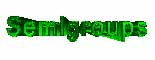Never thought that I would ever consider Galois descent of semigroup
coalgebras
but in preparing for my talks for the master-class it
came about naturally. Let A be a formally smooth algebra
(sometimes called a quasi-free algebra, I prefer the terminology
noncommutative curve) over an arbitrary base-field k. What, if
anything, can be said about the connected components of the affine
k-schemes rep(n,A) of n-dimensional representations
of A? If k is algebraically closed, then one can put a
commutative semigroup structure on the connected components induced by
the sum map

rep(n,A) x rep(m,A) -> rep(n + m,A)   :  (M,N)
-> M + N

as introduced and studied by Kent
Morrison
a long while ago. So what would be a natural substitute for
this if k is arbitrary? Well, define pi(n) to be the
maximal unramified sub k-algebra of k(rep(n,A)),
the coordinate ring of rep(n,A), then corresponding to the
sum-map above is a map

pi(n + m) -> pi(n) \\otimes
pi(m)

and these maps define on the graded
space

Pi(A) = pi(0) + pi(1) + pi(2) + ...

the
structure of a graded commutative k-coalgebra with
comultiplication

pi(n) -> sum(a + b=n) pi(a) \\otimes
pi(b)

The relevance of Pi(A) is that if we consider it
over the algebraic closure K of k we get the semigroup
coalgebra

K G  with  g -> sum(h.h\' = g) h \\otimes
h\'

where G is Morrison\’s connected component
semigroup. That is, Pi(A) is a k-form of this semigroup
coalgebra. Perhaps it is a good project for one of the students to work
this out in detail (and correct possible mistakes I made) and give some
concrete examples for formally smooth algebras A. If you know of
a reference on this, please let me know.